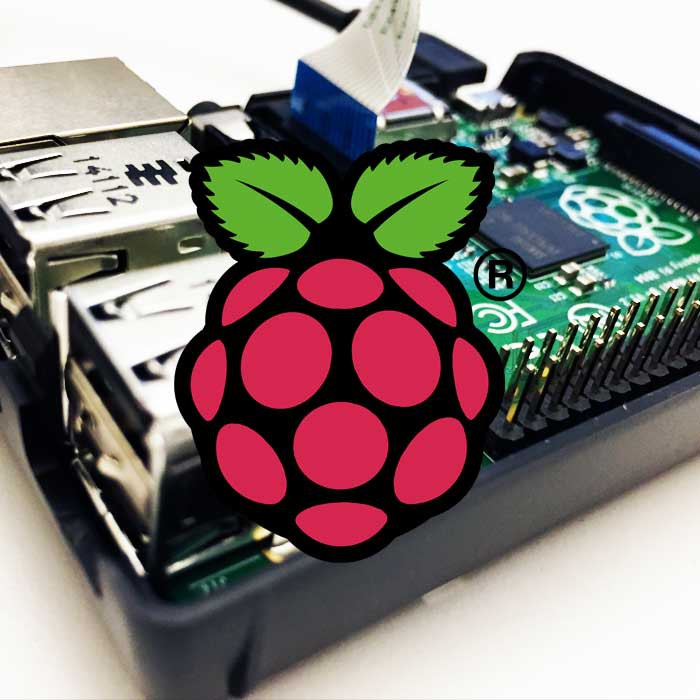## SmokeFX - An experiment with Bezier CurvesBy Andreas, 26 August, 2010

Since the dawn of the computer people has always created colourful art using curves. Either they have moved around on the screen or just being drawn with small adjustments creating mesmerizing patterns.
Being inspired by the latest PS3 boot menu I decided to create a nice looking (and dynamic) effect using Bezier curves. Here’s the treat:

Now, from start, there is a problem here. AS3 doesn’t support more than one control point (via the curveTo() method). To be a able to draw nice continuous curves we need 2 control points (Cubic Bezier).

Luckily I don’t have to worry about that as I could find this great class taking care of all that math I lost out as a very happy but maybe not so focused student. Wonderful! Most of the work done already, let’s put it to use!

I decided to divide the whole effect into 3 classes.

WaveData – Holding all the data about the wave including controlpoint-position. The Class is also controlling the logical animation of the controlpoints + the colour of the wave, using our beloved tweenengine TweenMax.

Wave – Being the DisplayObject that is the actual visual representation of the WaveData (being a SINGLE wave that is)

Canvas – This is the area that will be visual on the screen. All Waves are draw to the canvas and a few pretty effects are added to the canvas. We’ll get to that.

Now let’s get started with the WaveData:

```package
{
import com.greensock.TweenMax;
import com.greensock.easing.Sine;

import flash.geom.Point;

public class WaveData
{
public var controlPoints:int = 7
public var minWaveAlpha:Number = 0.09
public var maxWaveAlpha:Number = 0.3
public var waveAlpha:Number = .6;
public var lineWidth:Number = 0;
public var minSpeed:Number = 3;
public var maxSpeed:Number = 5;
public var color:int = 0;
public var aPoints:Array
private var alphaCount:int = 0;

// no constructor needed. Best practise?? Naah, maybe not but hey, I get to write a comment instead of a constructor.

// resets the wavedata and starts the animation of the controlpoints and the wavecolour.
public function reset()
{
var y:Number
var p:Point;
aPoints = [];

// create all controlpoints and place them within the radius. They all starts at x:0
for (var i:int = 0; i < controlPoints;i++)
{
p = new Point(0,y);
aPoints.push(p);
startAnim(p);
}
animColour();
animAlpha();
}

private function animColour()
{
var newColor = Math.random()*0xffffff;
TweenMax.to(this, 9, {hexColors:{color:newColor},onComplete:animColour,overwrite:false});
}

private function animAlpha()
{
alphaCount++;
if (alphaCount > 3)
{
TweenMax.to(this,2,{waveAlpha:0,overwrite:false});
}
else
{
TweenMax.to(this,2,{waveAlpha:Math.random()*(maxWaveAlpha-minWaveAlpha)+minWaveAlpha,onComplete:animAlpha,overwrite:false});
}
}

public function killWave()
{
TweenMax.killTweensOf(this);
for each(var p:Point in aPoints)
{
TweenMax.killTweensOf(p);
}
}

private function startAnim(p:Point)
{
// no, it's not an optimized way to find the point but it's a lab, remember?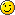var ip:int = aPoints.indexOf(p);
// x is being set "somewhat" around the section where it should be.
var newX:Number =  (ip==0)?0:((ip/(controlPoints-1)+(Math.random()*(1/(controlPoints-1))*3))-(1.5*(1/(controlPoints-1))));
// is the direction of the controlpoint going UP or DOWN?
if (p.y > 0) newY=-newY;
//animate!
TweenMax.to(p,(Math.random()*(maxSpeed-minSpeed))+minSpeed,{ease:Sine.easeInOut,y:newY,x:newX,onComplete:startAnim,onCompleteParams:[p]});
}
}
}
```

Pay attention to the fact that the controlpoints are just moving between 1 to -1 . This means that they are not having their physical width in here but will be multiplied later on in the Wave-class So let’s get going. Next class please!

```package
{
import flash.display.Sprite;
import flash.events.Event;
import flash.geom.Point;

public class Wave extends Sprite
{
public var waveWidth:Number = 650;
public var waveHeight:Number = 150;
public var data:WaveData

public function Wave()
{
super();
// creates data and starts the animation
data = new WaveData();
data.reset();
}

public function update()
{
// creating real coordinates from Wave-data controlpoints
var counter:int = 0;
var coord:Array = [];
for each(var p:Point in data.aPoints)
{
coord.push (new Point(p.x*waveWidth,p.y*waveHeight));;
}

// draw curve
graphics.clear();
graphics.lineStyle(data.lineWidth,data.color,data.waveAlpha);
CubicBezier.curveThroughPoints(graphics,coord);
}
}
}
```

Now the wave is clearly a fully animated wave. If you add this one to the stage you will see a thin line waving around.. but we want more, right? Next class please!

```package
{
import flash.display.Bitmap;
import flash.display.BitmapData;
import flash.display.BlendMode;
import flash.display.Sprite;
import flash.events.Event;
import flash.filters.BitmapFilter;
import flash.filters.BlurFilter;
import flash.geom.Matrix;
import flash.geom.Point;
import flash.geom.Rectangle;

public class Canvas extends Sprite
{
private var bmpd:BitmapData
private var aWaves:Array
private var w:int
private var h:int

public function Canvas(w:int = 900,h:int = 450)
{
super();

// set the width and height of the canvas
this.w = w;
this.h = h;

aWaves=[];

// adding a bitmap to stage
bmpd = new BitmapData(w,h,false,0);
var bmp:Bitmap = new Bitmap(bmpd);

}

{
// adding a wave to the canvas
aWaves.push(wave);
}

public function startDraw()
{
}

private function onTick(e:Event)
{
// each wave is now drawn onto the canvas using the ADD Blendmode.
for each ( var wave:Wave in aWaves)
{
wave.update();
var m:Matrix = new Matrix(1,0,0,1,26,bmpd.height*.5);
}

// the canvas is now blurred out giving a blurred AND transitionout effect.
var filter:BlurFilter = new BlurFilter(3,3);
bmpd.applyFilter(bmpd, new Rectangle(0,0,w,h),new Point(0,0), filter);

// the bitmapdata is now scrolled to the right giving a "moving feeling" to the effect.
bmpd.scroll(5,0)
}
}
}
```

Wow, amazing what a few postfilters can do. Just a blur and a pixelpush to the right does the trick. Now let’s put this all together in a Main class and try it out:

```package
{
import flash.display.Sprite;

public class Main extends Sprite
{
public function Main()
{
var c:Canvas = new Canvas();

for (var i:int = 0;i < 3;i++)
{
var w:Wave = new Wave();
}

c.startDraw();
}
}
}
```

## Memory game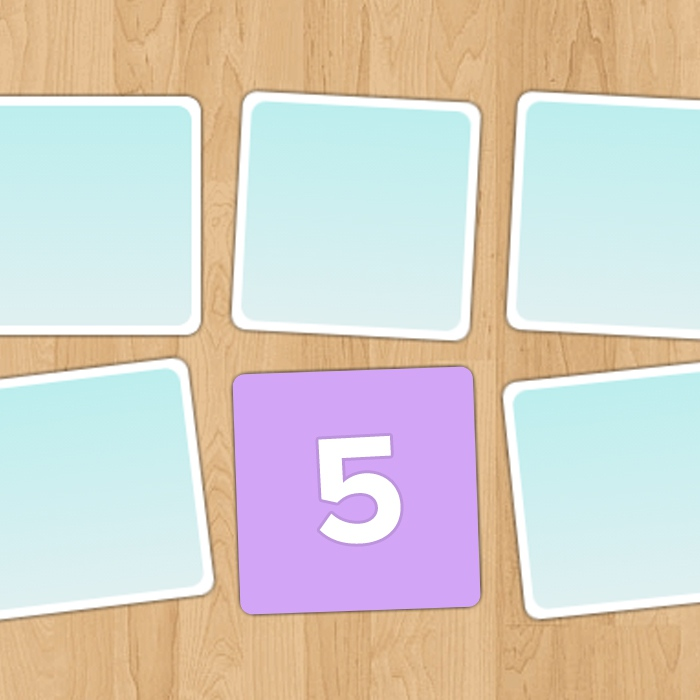## Air Hockey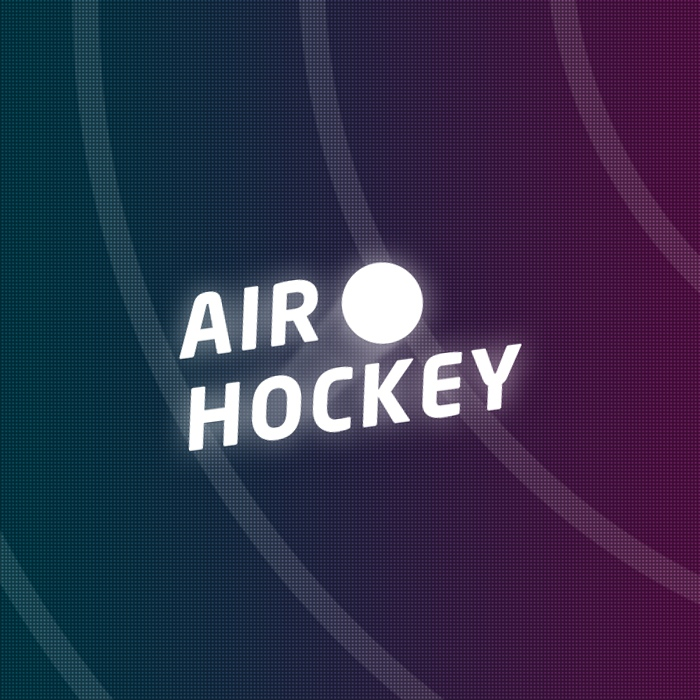## Particle system #1 - Basic particles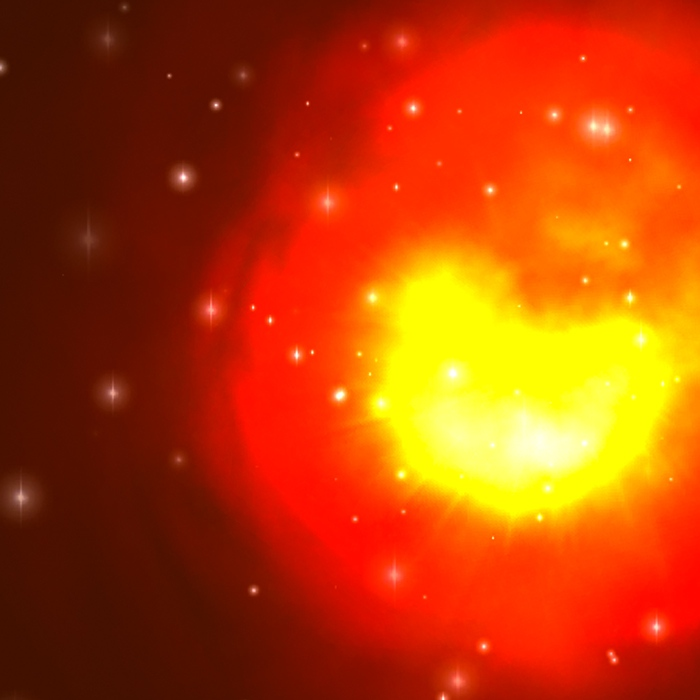## Rasberry Pi Image Streamer